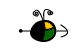Acidic and Basic Salt Solutions

Background Information

When certain soluble salts are dissolved in water the resulting solution is not neutral.  Depending on the composition of the salt (the ions which it is made up of) the solution will be either acidic or basic.  Soluble salts that contain anions derived from weak acids form solutions that are basic.  The anion is the conjugate base of a weak acid.  For example, the acetate ion is the conjugate base of acetic acid, a weak acid.  Therefore, a soluble acetate salt, such as sodium acetate will release acetate ions into the solution, which a few of these will interact with water, forming unionized acetic acid and the hydroxide ion.

NaCH3COO(s) --> Na+(aq) + CH3COO-(aq)

CH3COO-(aq) + H2O(l) --> CH3COOH(aq) + OH-

The latter reaction proceeds forward only to a small extent; the equilibrium constant K is very small.  The equilibrium expression for this reaction can be used to estimate the pH of the salt solution.  Since acetate functions as a weak base, the equilibrium constant is given the label Kb.

Soluble salts that contain cations derived from weak bases form solutions that are acidic.  The cation is the conjugate acid of a weak base.  For example, the ammonium ion is the conjugate acid of ammonia, a weak base.  Therefore, a soluble salt, such as ammonium chloride will release ammonium ions into the solution, which a few of these will interact with water, forming ammonia and the hydronium ion.

NH4Cl(s) --> NH4+(aq) + Cl-(aq)

NH4+ + H2O(l) --> NH3(aq) + H3O+(aq)

The latter reaction proceeds forward only to a small extent, the equilibrium constant K is very small.  The equilibrium expresion for this reaction can be used to estimate the pH of the salt solution.  Since the ammonium ion functions as a weak acid, the equilibrium constant is given the label Ka.

Top

Relationship between Ka and Kb of Conjugate Acid-Base Pairs

The relationship between Ka and Kb for any conjugate acid-base pairs is as follows:

(Ka)(Kb) = Kw

Where Ka is the ionization constant of the acid form of the pair, Kb is the ionization constant for the base form of the pair, and Kw is the ionization constant for water.  This equation is used to find either Ka or Kb when the other is known.

Example:  The Ka for acetic acid is 1.7 x 10-5.  What is the value of Kb for the acetate ion?

(1.7 x 10-5)(Kb) = 1 x 10-14
Kb = 5.9 x 10-10

Example:  The Kb for aniline is 3.8 x 10-10.  What is the value of Ka for the anilonium ion?

(Ka)(3.8 x 10-10) = 1 x 10-14
Ka = 2.6 x 10-5

Top

Calculating the pH of a Salt Solution

To calculate the pH of a salt solution one needs to know the concentration of the salt solution, whether the salt is an acidic, basic, or neutral salt, the equation for the interaction of the ion with the water, the equilibrium expression for this interaction and the Ka or Kb value.

Example:  Calculate the pH of a 0.500 M solution of KCN.  Ka for HCN is 5.8 x 10-10.

• First, write the equation for the dissolving process, and examine each ion formed to determine whether the salt is an acidic, basic, or neutral salt.
KCN(s) --> K+(aq) + CN-(aq)

K+ is a neutral ion and CN- is a basic ion.  KCN is a basic salt.

• Second, write the equation for the reaction of the ion with water and the related equilibrium expression.
CN-(aq) + H2O(l) --> HCN(aq) + OH-(aq)

Kb = [HCN][OH-]
[CN-]

• Third, use the given Ka for HCN to find the value of Kb for CN-.
(5.8 x 10-10)(Kb) = 1 x 10-14
Kb = 1.7 x 10-5
• Make an "ICE" chart to aid in the solution.  Let "x" represent the amount of CN- that interacts with the water.
 CN-(aq) HCN(aq) OH-(aq) Initial Concentration 0.500 M 0 M 0 M Change in Concentration - x + x + x Equilibrium Concentration 0.500 M - x x x
• Subsititute equilibrium values and the value for Kb to solve for x.
1.7 x 10-5 = (x)(x)/(0.500 - x)

We will make the assumption that since Kb is so small that the value for x will be very small as well, thus the term (0.500 - x) is equal to (0.500).

1.7 x 10-5 = x2/(0.500)
x = 2.9 x 10-3
• Determine the pH of the solution.  Since "x" represents the hydroxide ion concentration, we can convert it into pOH and than find the pH.
pOH = -log(2.9 x 10-3) = 2.54
pH = 14 - 2.54 = 11.46

Top

Example:  What would be the pH of a 0.200 M ammonium chloride solution?  Kb ammonia = 1.8 x 10-5.

NH4Cl(s) --> NH4+(aq) + Cl-(aq)

NH4+ is an acidic ion and Cl- is a neutral ion; solution will be acidic.

NH4+(aq) + H2O(l) --> NH3(aq) + H3O+(aq)

Ka = [NH3][H3O+]
[NH4+]

Ka = (1 x 10-14)/(1.8 x 10-5) = 5.6 x 10-10

Let x = the amount of NH4+ ion that reacts with the water.

 NH4+(aq) NH3(aq) H3O+(aq) Initial Concentration 0.200 M 0 0 Change in Concentration - x + x + x Equilibrium Concentration 0.200 M - x x x

5.6 x 10-10 = (x)(x)/(0.200 -x)

5.6 x 10-10 = x2/(0.200)

x = 1.1 x 10-5 M which is the H3O+ concentration.

pH = -log(1.1 x 10-5) = 4.98

Top

Example:  What is the pH of a 0.400 M KBr solution?

KBr(s) --> K+(aq) + Br-(aq)

K+ and Br- are both neutral ions.  The solution is neutral.

pH of the solution will be 7.

Top